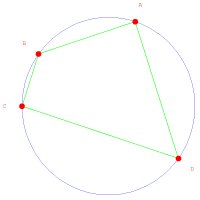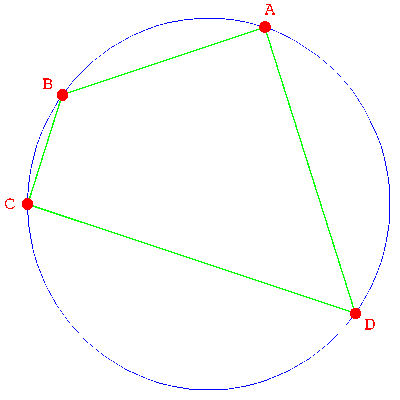## Exhibit: table of content

##### Oliver Knill, SciCtr 434, knill@math.harvard.eduSergey Sadov proved in 2004 the following theorem in elementary geometry.

 THEOREM: If ABCD is a quadrilateral on a circle, then ``` |AB| |BC| |CA| + |AC| |CD| |DA| = |BC| |CD| |DB| + |AB| |BD| |DA| ``` Proof. (This is a Mathematica version of a Maple proof found by Shalosh B. Ekhad, the computer collaborator of Doron Zeilberg at Rutgers university). ``` H[A_,B_]:=(A[]-B[])^2+(A[]-B[])^2; P[t_]:={t+1/t,(t-1/t)/I}/2; T[A_,B_,C_,D_]:=((A+B-C-D)^2-4*(A*B+C*D))^2-64*A*B*C*D; U[A_,B_,C_]:=H[A,B]*H[B,C]*H[C,A]; K={P[t],P[t],P[t],P[t]}; S=T[U[P[t],P[t],P[t]],U[P[t],P[t],P[t]], U[P[t],P[t],P[t]],U[P[t],P[t],P[t]]]==0; Simplify[S] ``` Side remark: Ekhads Maple Proof uses a 360 Character Maple code, which proves it in 0.05 seconds. The above Mathematica code has 336 characters and needed 0.01 seconds to complete on a Pentium D 840. Remark added Mai 4, 2007: The swiss mathematician Roland Stärk informed us of an elegant proof of Sadovs theorem: a triangle A,B,C with sides a,b,c has area ``` A = a b sin(gamma)/2 = abc/(4r) ``` if r is the radius of the circle through A,B,C. [This formula uses a basic property of the cross product and the sin-theorem sin(gamma)/c = 2/r, applied to the right angle triangle A,(A+B)/2,(A+B+C)/3.] Sadovs formula just expresses the area of the quadrilateral in two different ways. Stärk wrote us that conversely, the formula |AB| |BC| |CA| + |AC| |CD| |DA| = |BC| |CD| |DB| + |AB| |BD| |DA| implies that the quadrilateral triangle is on a circle but that this is harder to prove.

 Postscript: Sergey Sadov writes us in February 2008: "For the sake of fairness, this theorem should not be attributed to me. Its history, to the best of my knowlege, is sketched in his presentation [PDF].